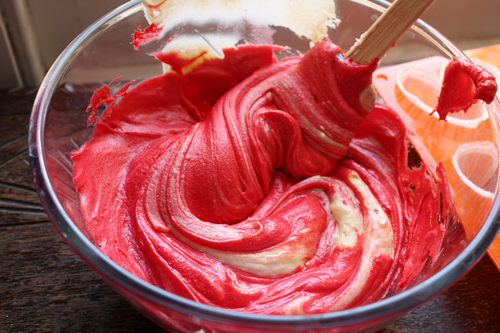grade 10 CHEM test for exam

good to study for the exam grade 10 chemistry unit ... .. . .. ... . . .

-4
-2
-1
-3
+2

which is a balanced equation of P4 + O2 = P2O5

2P4 + 3O2 = 4P2O5
P4 + 5O2 = 2P2O5
5P + 1O2 = 3P2O5

what is an example of a skeleton equation?

Select the two correct answers
NaCl + 2Na + Cl
Cl2 + 2NaBr = Br2 + 2NaCl
2NaCl = 2Na + 2 CL

example of single displacement

AB + CD = AD + CB
A + BC = AC + B

sign of a chemical change

Select the five correct answers
new smell
new color
impossible to reverse
precipitate is formed
substance breaks down
bubbles form
heat is given off

Na3N
Na2N3
NaN2

true
false

pure substances break into

Select the three correct answers
non metal
metals
metalloids
acids
bases
neutral